# 高阶非线性减振系统的主动控制的研究Study on the Active Control of Vertical Nonlinear Suspension System

• 全文下载: PDF(1043KB)    PP.79-87   DOI: 10.12677/OJAV.2018.63010
• 下载量: 539  浏览量: 1,380   国家自然科学基金支持

The vehicle suspension system can be simplified into a single-degree-of-freedom vibration model. Five nonlinear stiffness factors are considered in this model, and a time-delay damper is used to control its vertical vibration. Using multi-scale analysis, the theoretical approximate solution of the system is obtained. The effects of nonlinear stiffness coefficient, damping coefficient, feedback gain coefficient and time delay on vertical vibration reduction are studied, and the regularity is analyzed, which can provide theoretical guidance for vibration reduction projects in engineering applications.

1. 引言

2. 力学模型

$m\stackrel{¨}{x}\left(t\right)+kx\left(t\right)+\delta {x}^{5}\left(t\right)+c\stackrel{˙}{x}\left(t\right)+{f}_{sc}\stackrel{¨}{x}\left(t-\tau \right)={F}_{0}\mathrm{cos}\left(wt\right)$ (1)Figure 1. Illustration of model

3. 摄动分析

$\frac{\text{d}x}{\text{d}t}=\frac{w{x}_{c}}{\Omega }\cdot \frac{\text{d}y}{\text{d}T}$$\frac{{\text{d}}^{2}x}{\text{d}{t}^{2}}=\frac{{w}^{2}{x}_{c}}{{\Omega }^{2}}\cdot \frac{{\text{d}}^{2}y}{{\text{d}}^{2}T}$$\frac{{\text{d}}^{2}x\left(t-\tau \right)}{\text{d}{t}^{2}}=\frac{{w}^{2}{x}_{c}}{{\Omega }^{2}}\cdot \frac{{\text{d}}^{2}y\left(T-{\tau }_{c}\right)}{\text{d}{T}^{2}}$ (2)

$y=\frac{x}{{x}_{c}}$$T=\frac{w}{\Omega }t$${\tau }_{c}=\frac{w}{\Omega }\tau$${w}_{0}=\sqrt{\frac{k{\Omega }^{2}}{m{w}^{2}}}$${\xi }_{1}=\frac{\delta {x}_{c}^{4}{\Omega }^{2}}{m{w}^{2}}$${\xi }_{2}=\frac{c\Omega }{mw}$${f}_{s}=\frac{{f}_{sc}}{{m}_{c}}$$F=\frac{{F}_{0}{\Omega }^{2}}{m{x}_{c}{w}^{2}}$ (3)

$\stackrel{¨}{y}\left(T\right)+{w}_{0}^{2}y\left(T\right)+{\xi }_{1}{y}^{5}\left(T\right)+{\xi }_{2}\stackrel{˙}{y}\left(T\right)+{f}_{s}\stackrel{¨}{y}\left(T-{\tau }_{c}\right)=F\mathrm{cos}\left(\Omega T\right)$ (4)

${\xi }_{1}=\epsilon {\stackrel{^}{\xi }}_{1}$${\xi }_{2}=\epsilon {\stackrel{^}{\xi }}_{2}$${f}_{s}=\epsilon {\stackrel{^}{f}}_{s}$$F=\epsilon \stackrel{^}{f}$$\tau ={\tau }_{c}=\stackrel{^}{\tau }$${y}_{\tau }=y\left(T-\stackrel{^}{\tau }\right)$$\Omega ={w}_{0}+\epsilon \sigma$

$\stackrel{¨}{y}\left(T\right)+{w}_{0}^{2}y\left(T\right)=\epsilon \left[-{\stackrel{^}{\xi }}_{1}{y}^{5}\left(T\right)-{\stackrel{^}{\xi }}_{2}\stackrel{˙}{y}\left(T\right)-{\stackrel{^}{f}}_{s}{\stackrel{˙}{y}}_{\stackrel{^}{\tau }}+\stackrel{^}{f}\mathrm{cos}\left(\Omega T\right)\right]$ (5)

$y\left(T,\epsilon \right)={y}_{0}\left({T}_{0},{T}_{1}\right)+\epsilon {y}_{1}\left({T}_{0},{T}_{1}\right)$${y}_{\stackrel{^}{\tau }}\left(T,\epsilon \right)={y}_{0\stackrel{^}{\tau }}\left({T}_{0},{T}_{1}\right)+\epsilon {y}_{1\stackrel{^}{\tau }}\left({T}_{0},{T}_{1}\right)$ (6)

${\epsilon }^{0}:{D}_{0}^{2}{y}_{0}+{\omega }_{0}^{2}{y}_{0}=0$ (7)

${\epsilon }^{1}:{D}_{0}^{2}{y}_{1}+{w}_{0}^{2}{y}_{1}=-2{D}_{0}{D}_{1}{y}_{0}+\left[-{\stackrel{^}{\xi }}_{1}{y}_{0}^{5}-{\stackrel{^}{\xi }}_{2}{D}_{0}{y}_{0}-{\stackrel{^}{f}}_{s}{\stackrel{˙}{y}}_{0\stackrel{^}{\tau }}+\stackrel{^}{f}\mathrm{cos}\left({w}_{0}{T}_{0}+\sigma {T}_{1}\right)\right]$ (8)

${y}_{0}=a\left({T}_{1}\right)\mathrm{cos}\left[{w}_{0}{T}_{0}+\phi \left({T}_{1}\right)\right]=A\left({T}_{1}\right){\text{e}}^{i{w}_{0}{T}_{0}}+cc$ (9)

$\stackrel{^}{f}\mathrm{cos}\left({w}_{0}{T}_{0}+\sigma {T}_{1}\right)=\frac{1}{2}\stackrel{^}{f}{\text{e}}^{i\left({w}_{0}{T}_{0}+\sigma {T}_{1}\right)}+cc$ (10)

${y}_{0\tau }={A}_{\tau }\left({T}_{1}\right){\text{e}}^{i{w}_{0}\left({T}_{0}-\tau \right)}+cc$ (11)

${A}_{\tau }=A\left({T}_{1}\right)-\epsilon \tau {A}^{\prime }\left({T}_{1}\right)+\frac{{\left(\epsilon \tau \right)}^{2}}{2}{A}^{″}\left({T}_{1}\right)+\cdots$ (12)

 (13)

$-i2{w}_{0}{D}_{1}A-10{\stackrel{^}{\xi }}_{1}{A}^{3}{\stackrel{¯}{A}}^{2}-i{w}_{0}{\stackrel{^}{\xi }}_{2}A-i{w}_{0}{\stackrel{^}{f}}_{s}A{\text{e}}^{-i{w}_{0}\stackrel{^}{\tau }}+\frac{\stackrel{^}{f}}{2}{\text{e}}^{i\sigma {T}_{1}}=0$ (14)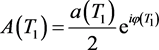代入(14)

$-i{w}_{0}{D}_{1}a+a{w}_{0}{D}_{1}\phi -\frac{5}{16}{\stackrel{^}{\xi }}_{1}{a}^{5}-i{w}_{0}{\stackrel{^}{\xi }}_{2}\frac{a}{2}-i{w}_{0}{\stackrel{^}{f}}_{s}\frac{a}{2}{\text{e}}^{-i{w}_{0}\stackrel{^}{\tau }}+\frac{\stackrel{^}{f}}{2}{\text{e}}^{i\left(\sigma {T}_{1}-\phi \right)}=0$ (15)

${D}_{1}a=-\frac{a}{2}{\stackrel{^}{\xi }}_{2}-{\stackrel{^}{f}}_{s}\frac{a}{2}\mathrm{cos}\left({w}_{0}\stackrel{^}{\tau }\right)+\frac{\stackrel{^}{f}}{2{w}_{0}}\mathrm{sin}\left(\sigma {T}_{1}-\phi \right)$ (16)

$a{D}_{1}\phi =\frac{5}{16{w}_{0}}{\stackrel{^}{\xi }}_{1}{a}^{5}+{\stackrel{^}{f}}_{s}\frac{a}{2}\mathrm{sin}\left({w}_{0}\tau \right)-\frac{\stackrel{^}{f}}{2{w}_{0}}\mathrm{cos}\left(\sigma {T}_{1}-\phi \right)$ (17)

$\varphi =\sigma {T}_{1}-\phi$ ，则上式为：

 (18)

$a\sigma -a{D}_{1}\varphi =\frac{5}{16{w}_{0}}{\stackrel{^}{\xi }}_{1}{a}^{5}+{\stackrel{^}{f}}_{s}\frac{a}{2}\mathrm{sin}\left({w}_{0}\stackrel{^}{\tau }\right)-\frac{\stackrel{^}{f}}{2{w}_{0}}\mathrm{cos}\varphi$ (19)

$\frac{\stackrel{^}{f}}{2}\mathrm{sin}\varphi =\frac{a}{2}{w}_{0}{\stackrel{^}{\xi }}_{2}+{\stackrel{^}{f}}_{s}{w}_{0}\frac{a}{2}\mathrm{cos}\left({w}_{0}\stackrel{^}{\tau }\right)$ (20)

$\frac{\stackrel{^}{f}}{2}\mathrm{cos}\varphi =\frac{5}{16}{\stackrel{^}{\xi }}_{1}{a}^{5}+{\stackrel{^}{f}}_{s}{w}_{0}\frac{a}{2}\mathrm{sin}\left({w}_{0}\stackrel{^}{\tau }\right)-a\sigma {w}_{0}$ (21)

$\frac{{\stackrel{^}{f}}^{2}}{4}={\left[\frac{a}{2}{w}_{0}{\stackrel{^}{\xi }}_{2}+{\stackrel{^}{f}}_{s}{w}_{0}\frac{a}{2}\mathrm{cos}\left({w}_{0}\stackrel{^}{\tau }\right)\right]}^{2}+{\left[\frac{5}{16}{\stackrel{^}{\xi }}_{1}{a}^{5}+{\stackrel{^}{f}}_{s}{w}_{0}\frac{a}{2}\mathrm{sin}\left({w}_{0}\stackrel{^}{\tau }\right)-a\sigma {w}_{0}\right]}^{2}$ (22)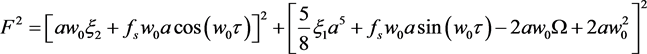(23)

4. 系统参数对减振性能的影响

${F}^{2}={\left[a{w}_{0}{\xi }_{2}\right]}^{2}+{\left[\frac{5}{8}{\xi }_{1}{a}^{5}-2a{w}_{0}\Omega +2a{w}_{0}^{2}\right]}^{2}$ (24)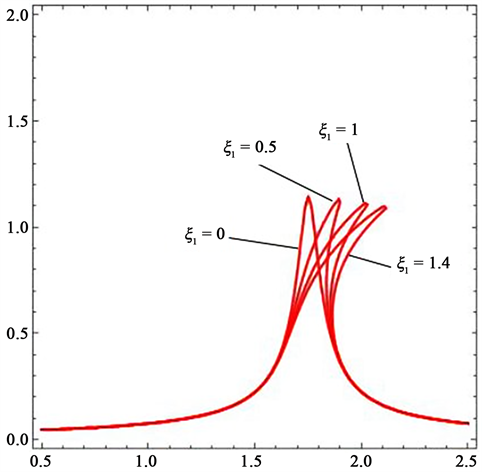Figure 2. Stiffness versus amplitude

5. 系统的稳定性与分岔

${S}_{1}=\frac{\partial {f}_{1}}{\partial a}=-\frac{{\stackrel{^}{\xi }}_{2}}{2}-\frac{{\stackrel{^}{f}}_{s}}{2}\mathrm{cos}\left({w}_{0}\stackrel{^}{\tau }\right)$${S}_{2}=\frac{\partial {f}_{1}}{\partial \varphi }=\frac{\stackrel{^}{f}}{2{w}_{0}}\mathrm{cos}\varphi =\frac{5}{16{w}_{0}}{\stackrel{^}{\xi }}_{1}{a}^{5}+{\stackrel{^}{f}}_{s}\frac{a}{2}\mathrm{sin}\left({w}_{0}\stackrel{^}{\tau }\right)-a\sigma$

${S}_{3}=\frac{\partial {f}_{2}}{\partial a}=\frac{1}{a}\left[\sigma -\frac{25}{16{w}_{0}}{\stackrel{^}{\xi }}_{1}{a}^{4}-{\stackrel{^}{f}}_{s}\frac{1}{2}\mathrm{sin}\left({w}_{0}\stackrel{^}{\tau }\right)\right]$${S}_{4}=\frac{\partial {f}_{2}}{\partial \varphi }=\frac{\stackrel{^}{f}}{2{w}_{0}}\mathrm{sin}\varphi =-\frac{1}{2}{\stackrel{^}{\xi }}_{2}-{\stackrel{^}{f}}_{s}\frac{1}{2}\mathrm{cos}\left({w}_{0}\stackrel{^}{\tau }\right)$

$cr=\left[\frac{5}{16{w}_{0}}{\stackrel{^}{\xi }}_{1}{a}^{4}+{\stackrel{^}{f}}_{s}\frac{1}{2}\mathrm{sin}\left({w}_{0}\stackrel{^}{\tau }\right)-\sigma \right]\left[\sigma -\frac{25}{16{w}_{0}}{\stackrel{^}{\xi }}_{1}{a}^{4}-{\stackrel{^}{f}}_{s}\frac{1}{2}\mathrm{sin}\left({w}_{0}\stackrel{^}{\tau }\right)\right]$

$\mathrm{det}\left[\begin{array}{cc}{S}_{1}-\lambda & {S}_{2}\\ {S}_{3}& {S}_{4}-\lambda \end{array}\right]=0$ (25)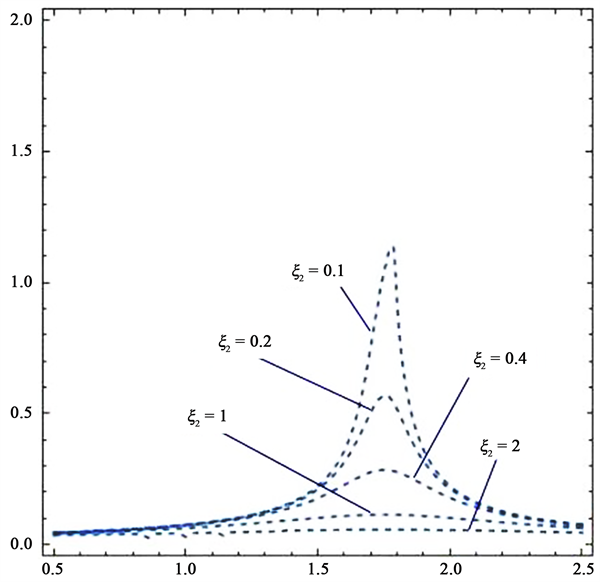Figure 3. Damping versus amplitude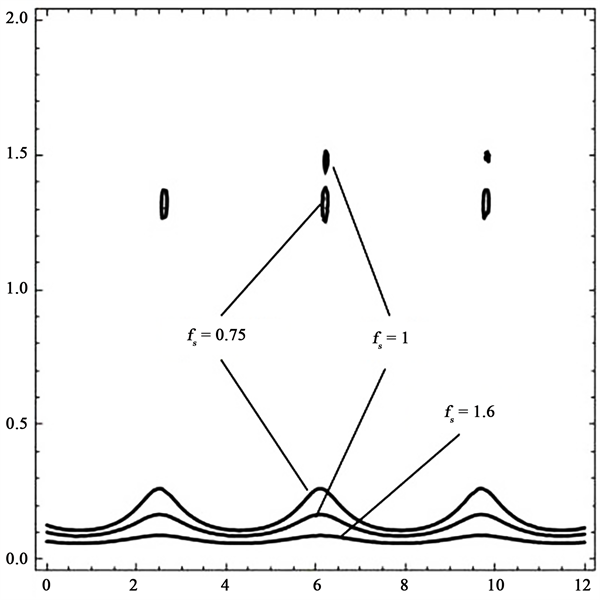Figure 4. Time delay versus amplitude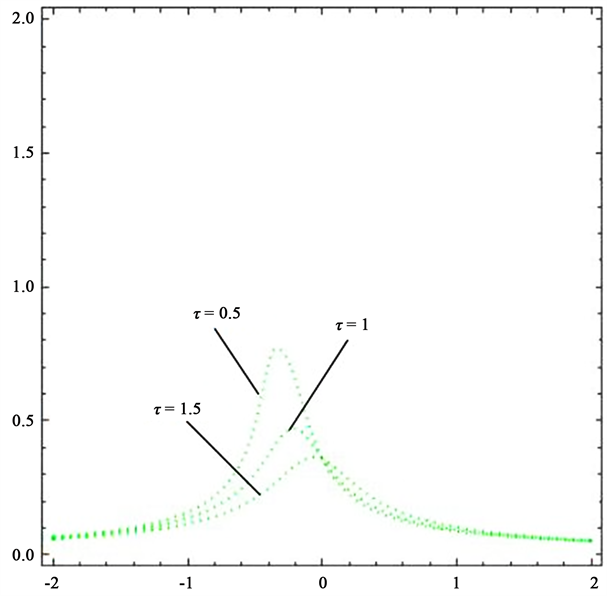Figure 5. Feedback gain versus amplitude

$B=\left[{\stackrel{^}{\xi }}_{2}+{\stackrel{^}{f}}_{s}\mathrm{cos}\left({w}_{0}\stackrel{^}{\tau }\right)\right]>0$

$A={\left[\frac{{\stackrel{^}{\xi }}_{2}}{2}+\frac{{\stackrel{^}{f}}_{s}}{2}\mathrm{cos}\left({w}_{0}\stackrel{^}{\tau }\right)\right]}^{2}-\left[\frac{5}{16{w}_{0}}{\stackrel{^}{\xi }}_{1}{a}^{4}+{\stackrel{^}{f}}_{s}\frac{1}{2}\mathrm{sin}\left({w}_{0}\stackrel{^}{\tau }\right)-\sigma \right]\left[\sigma -\frac{25}{16{w}_{0}}{\stackrel{^}{\xi }}_{1}{a}^{4}-{\stackrel{^}{f}}_{s}\frac{1}{2}\mathrm{sin}\left({w}_{0}\stackrel{^}{\tau }\right)\right]>0$

6. 结论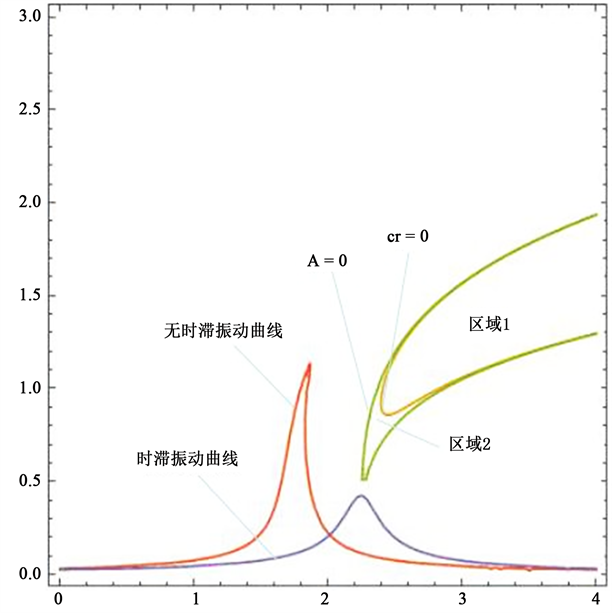Figure 6. Stability domain versus oscillating curve

NOTES

*通讯作者。

  胡海岩, 王在华. 非线性时滞动力系统的研究进展[J]. 力学进展, 1999, 29(4): 501-512.  陈泽深, 王成国. 车辆-轨道系统高中低频动力学模型的理论特征及其应用范围的研究[J]. 中国铁道科学, 2004, 25(4): 1-10.  金景云. 高速列车用油压减振器的研究[D]: [硕士学位论文]. 上海: 上海交通大学, 2000.  Margolis, D.L., Tyle, J.L. and Hrovat, D. (1997) Heave Mode Dynamics of a Tracked Air Custion Vehicle with Semi-Active Airbag Secondary Suspension. ASME Journal of Dynamic Systems Measurement and Control, 119, 399-407. https://doi.org/10.1115/1.3426956  Elmadany, M.M. and Abduljabbar, Z. (1991) Alternative Control Laws for Active and Semi-Active Automotive Suspensions—A Comparative Study. Computers & Structures, 39, 3-4.  陈龙, 汪若尘, 江浩斌, 等. 含时滞半主动悬架及其控制系统[J]. 机械工程学报, 2006, 42(1): 130-133.  金善玉. 高速列车转向架自振频率测试方法研究[D]: [硕士学位论文]. 长春: 吉林大学, 2012.  马新娜, 杨绍普. 时滞在磁流变主动控制系统中的影响[J]. 动力学与控制学报, 2012, 10(3): 228-233.  朱茂飞, 陈无畏, 祝辉. 基于磁流变减振器的半主动悬架时滞变结构控制[J]. 机械工程学报, 2010, 46(12): 113-120.  赵剡水, 周孔亢, 李仲兴, 等. 磁流变减振器半主动悬架的系统时滞[J]. 机械工程学报, 2009, 45(7): 221-227.